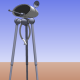## GIRC Distance CommandsIn the above illustration, the first Command Element has a command code of 44, which tells us that it is a Move command, and that we are to move 360 plus whatever is in the second Command Element minus 33. Why this bit of complicated arithmetic?

Ironically, to simplify things. First of all, I don’t want to send raw segment coordinate data to the microcontroller, because then to do the math calculations in the microcontroller I would have to load the floating point trig libraries into it and that would take up a lot of memory that I may want for something else. Second, I want to limit the data I send over the serial port to the printable ASCII character set between 33 and 127 in byte values.

So I do all the heavy math in the computer and then encode the path/segment turn-and-move data into a format of a series of bytes ranging in value from 33 to 127. The convoluted but simple arithmetic can then be done by the microcontroller to convert back to the actual turns and moves.